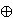### Mirela Jukić Bokun

Department of Mathematics, University of Osijek, Trg Ljudevita Gaja 6, 31 000 Osijek, Croatia
e-mail: mirela@mathos.hr

Abstract.   For each of the torsion groups Z/2ZZ/10Z, Z/2ZZ/12Z, Z/15Z we find the quadratic field with the smallest absolute value of its discriminant such that there exists an elliptic curve with that torsion and positive rank. For the torsion groups Z/11Z, Z/14Z we solve the analogous problem after assuming the Parity conjecture.

2010 Mathematics Subject Classification.   11G05, 14H52, 11R11.

Key words and phrases.   Elliptic curve, torsion group, rank, quadratic field.

Full text (PDF) (free access)

DOI: 10.3336/gm.47.2.04

References:

1. W. Bosma, J. Cannon and C. Playoust, The Magma algebra system. I. The user language, J. Symbolic Comput. 24 (1997), 235-265.
Magma
CrossRef

2. J. Bosman, P. Bruin, A. Dujella and F. Najman, Ranks of elliptic curves with prescribed torsion over number fields, preprint.

3. J. E. Cremona, Algorithms for Modular Elliptic Curves, Cambridge University Press, 1997.
MathSciNet

4. T. Dokchitser, Notes on the Parity Conjecture, to appear in a CRM Advanced Courses volume, Birkhauser.

5. A. Dujella and M. Jukić Bokun, On the rank of elliptic curves over Q(i) with torsion group Z/4Z × Z/4Z, Proc. Japan Acad. Ser. A Math. Sci. 86 (2010), 93-96.
MathSciNet     CrossRef

6. M. Jukić Bokun, On the rank of elliptic curves over Q((-3)1/2) with torsion groups Z/3Z × Z/3Z and Z/3Z × Z/6Z, Proc. Japan Acad. Ser. A Math. Sci. 87 (2011), 61-64.
MathSciNet     CrossRef

7. S. Kamienny, Torsion points on elliptic curves and q-coefficients of modular forms, Invent. Math. 109 (1992), 221-229.
MathSciNet     CrossRef

8. S. Kamienny and F. Najman, Torsion groups of elliptic curves over quadratic fields, Acta. Arith. 152 (2012), 291-305.
MathSciNet     CrossRef

9. M. A. Kenku and F. Momose, Torsion points on elliptic curves defined over quadratic fields, Nagoya Math. J. 109 (1988), 125-149.
MathSciNet     CrossRef

10. L. Kulesz, Families of elliptic curves of high rank with nontrivial torsion group over Q, Acta Arith. 108 (2003), 339-356.
MathSciNet     CrossRef

11. B. Mazur, Rational isogenies of prime degree, Invent. Math. 44 (1978), 129-162.
MathSciNet     CrossRef

12. F. P. Rabarison, Structure de torsion des courbes elliptiques sur les corps quadratiques, Acta Arith. 144 (2010), 17-52.
MathSciNet     CrossRef

13. D. Simon, Le fichier gp.